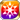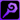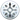# Lurking at the Carnival

Type
Equipment
Rank
5★
Max level
40
Enhancer cost
2000
Enhancer EXP
20000
Vision Clear Reward
Source
Time Limited Summon (Halloween)
Release dates
• JP: 2019-10-17
• GL: 2020-10-15
AlchemistCodeDBHalloween is a wonderful thing. I must say that I am unsure as to why my choice of Halloween costume is a problem, however. After all, Halloween is precisely the occasion to wear garments as gorgeous and gaudy as one pleases. Oh! Melda appears to be enjoying herself. Most excellent. She seems to be getting along rather well with Miss Cheryl, if the bold compliment she just paid her is anything to go by. Miss Cheryl appears rather embarrassed, but not displeased, as she responds, "Wh-why would you say something like that all of a sudden? I will not penalize you for that nonsensical statement, but really..." I am most pleased. As I always say, Halloween is a season to be enjoyed with one's friends! (Alexis' internal monologue as he entertains some children)

## Stats

#### Simple stats

TypeLvl 1Lvl 30Lvl 40
MATK+10+24+30

LevelMATK
1+10
2+10
3+11
4+11
5+12
6+12
7+13
8+13
9+14
10+14
LevelMATK
11+15
12+15
13+16
14+16
15+17
16+17
17+18
18+18
19+19
20+19
LevelMATK
21+20
22+20
23+21
24+21
25+22
26+22
27+23
28+23
29+24
30+24
LevelMATK
31+25
32+25
33+26
34+26
35+27
36+27
37+28
38+28
39+29
40+30

## Group skills

### Slothstein's Dark Essence

#### Simple stats

TypeLvl 1Lvl 30Lvl 40
Strong vs Dark+5+13+16
MDEF+13+33+40

#### Detailed stats

LevelStrong vs DarkMDEF
1+5+13
2+5+13
3+5+14
4+5+15
5+6+15
6+6+16
7+6+17
8+6+17
9+7+18
10+7+19
LevelStrong vs DarkMDEF
11+7+19
12+8+20
13+8+21
14+8+22
15+8+22
16+9+23
17+9+24
18+9+24
19+10+25
20+10+26
LevelStrong vs DarkMDEF
21+10+26
22+10+27
23+11+28
24+11+28
25+11+29
26+12+30
27+12+31
28+12+31
29+12+32
30+13+33
LevelStrong vs DarkMDEF
31+13+33
32+13+34
33+14+35
34+14+35
35+14+36
36+14+37
37+15+37
38+15+38
39+15+39
40+16+40

#### Limit Break

TypeLB1LB2LB3LB4LB5
Strong vs Dark+2+4+6+8+10
MDEF+5+9+14+19+24

### Slothstein's Dark Essence

#### Simple stats

TypeLvl 1Lvl 30Lvl 40
Strong vs Dark+5+13+16
MDEF+13+33+40

#### Detailed stats

LevelStrong vs DarkMDEF
1+5+13
2+5+13
3+5+14
4+5+15
5+6+15
6+6+16
7+6+17
8+6+17
9+7+18
10+7+19
LevelStrong vs DarkMDEF
11+7+19
12+8+20
13+8+21
14+8+22
15+8+22
16+9+23
17+9+24
18+9+24
19+10+25
20+10+26
LevelStrong vs DarkMDEF
21+10+26
22+10+27
23+11+28
24+11+28
25+11+29
26+12+30
27+12+31
28+12+31
29+12+32
30+13+33
LevelStrong vs DarkMDEF
31+13+33
32+13+34
33+14+35
34+14+35
35+14+36
36+14+37
37+15+37
38+15+38
39+15+39
40+16+40

#### Limit Break

TypeLB1LB2LB3LB4LB5
Strong vs Dark+2+4+6+8+10
MDEF+5+9+14+19+24

### Protector of the Harvest

#### Simple stats

TypeLvl 1Lvl 30Lvl 40
MATK+20+49+60
Magic Res+2+6+8

#### Detailed stats

LevelMATKMagic Res
1+20+2
2+21+2
3+22+2
4+23+2
5+24+2
6+25+2
7+26+2
8+27+3
9+28+3
10+29+3
LevelMATKMagic Res
11+30+3
12+31+3
13+32+3
14+33+4
15+34+4
16+35+4
17+36+4
18+37+4
19+38+4
20+39+4
LevelMATKMagic Res
21+40+5
22+41+5
23+42+5
24+43+5
25+44+5
26+45+5
27+46+6
28+47+6
29+48+6
30+49+6
LevelMATKMagic Res
31+50+6
32+51+6
33+52+6
34+53+7
35+54+7
36+55+7
37+56+7
38+57+7
39+58+7
40+60+8

#### Limit Break

TypeLB1LB2LB3LB4LB5
MATK+7+14+21+28+36
Magic Res+1+2+3+4+5

### Arbiter of Magic

#### Simple stats

TypeLvl 1Lvl 30Lvl 40
HP+100+323+400
Magic ATK Power+3+8+10
Silence Res+13+33+40

#### Detailed stats

LevelHPMagic ATK PowerSilence Res
1+100+3+13
2+107+3+13
3+115+3+14
4+123+3+15
5+130+3+15
6+138+3+16
7+146+4+17
8+153+4+17
9+161+4+18
10+169+4+19
LevelHPMagic ATK PowerSilence Res
11+176+4+19
12+184+4+20
13+192+5+21
14+200+5+22
15+207+5+22
16+215+5+23
17+223+5+24
18+230+6+24
19+238+6+25
20+246+6+26
LevelHPMagic ATK PowerSilence Res
21+253+6+26
22+261+6+27
23+269+6+28
24+276+7+28
25+284+7+29
26+292+7+30
27+300+7+31
28+307+7+31
29+315+8+32
30+323+8+33
LevelHPMagic ATK PowerSilence Res
31+330+8+33
32+338+8+34
33+346+8+35
34+353+8+35
35+361+9+36
36+369+9+37
37+376+9+37
38+384+9+38
39+392+9+39
40+400+10+40

#### Limit Break

TypeLB1LB2LB3LB4LB5
HP+48+96+144+192+240
Magic ATK Power+1+2+3+4+6
Silence Res+5+9+14+19+24

TypeLB5
Light+10

## Vision abilities

### Limit Reached Bonus

#### Ability base

• Slot:Support

Revealed after Limit Reached. Raises AGI after appearing on map.

#### Ability max

• Slot:Action

Raises AGI after appearing on map.

#### Ability base

• Slot:Action
• DMG Type:Magical
• ATK Type:Magic
• Element:Light

Light Mag ATK on units within area [Range: 3, Area: Diamond (5), Height Range: 2]

Skill Effect
TypeMinMax
Attack100%140%
• Charges: 2
• Jewel Cost: 55
• Height: 2
• Select Range: Diamond
• Range: 0-3
• Select Scope: Diamond
• Scope: 2

#### Ability max

• Slot:Action
• DMG Type:Magical
• ATK Type:Magic
• Element:Light

Light Mag ATK on units within area [Range: 4, Area: Diamond (5), Height Range: 2]

Skill Effect
TypeMinMax
Attack182%182%
• Charges: 2
• Jewel Cost: 55
• Height: 2
• Select Range: Diamond
• Range: 0-4
• Select Scope: Diamond
• Scope: 2